# Punctured Convolutional Coding in Simulink

This example simulates a punctured coding system that uses rate 1/2 convolutional encoding and Viterbi decoding. The complexity of a Viterbi decoder increases rapidly with the code rate. The puncturing technique enables encoding and decoding of higher rate codes by using standard lower rate coders.

The cm_punct_conv_code model transmits a convolutionally encoded BPSK signal through an AWGN channel, demodulates the received signal, and then performs Viterbi decoding to recover the uncoded signal. To compute the error rate, the model compares the original % signal and the decoded signal.The model uses the PreloadFcn callback function to set these workspace variables for initialization of block parameters:

puncvec = [1;1;0;1;1;0]; EsN0dB = 2; traceback = 96; % Viterbi traceback depth

The blocks in this model perform these operations:

• Bernoulli Binary Generator — Sets Sample per frames to 3. The block creates a sequence of random bits outputting three samples per frame at each sample time.

• Convolutional Encoder — Uses the default setting for Trellis structure, selects Puncture code, and sets Puncture vector to the workspace variable puncvec. The block encodes frames of data by puncturing a rate 1/2, constraint length 7 convolutional code to a rate 3/4 code. The puncture vector specified by puncvec is the optimal puncture vector for the rate 1/2, constraint length 7 convolutional code. A 1 in the puncture vector indicates that the bit in the corresponding position of the coded vector is sent to the output vector, while a 0 indicates that the bit is removed. For the configured encoder, coded bits in positions 1, 2, 4, and 5 are transmitted, while bits in positions 3 and 6 are removed. The rate 3/4 code means that for every 3 bits of input, the punctured code generates 4 bits of output.

• BPSK Modulator Baseband — Modulates the encoded message using default parameter values.

• AWGN Channel — Sets Mode to Signal to noise ratio (Es/No) and sets Es/No (dB) to the workspace variable EsN0dB. Since the modulator block generates unit power signals, Input signal power, referenced to 1 ohm (watts) keeps its default value of 1.

• Viterbi Decoder — Uses settings for Trellis structure, Punctured code, and Puncture vector that align with the Convolutional Encoder block. The block sets Decision type to Unquantized and Traceback depth to the traceback workspace variable. To decode the specified convolutional code without puncturing the code, a traceback depth of 40 is sufficient. However, to give the decoder enough data to resolve the ambiguities introduced by the punctures, the block uses a traceback depth of 96 to decode the punctured code. Similar to the convolutional encoder, the puncture vector for the decoder indicates the locations of the punctures. For the decoder, the locations are bits to ignore in the decoding process because the punctured bits are not transmitted and there is no information to indicate their values. Each 1 in the puncture vector indicates a transmitted bit, and each 0 indicates a punctured bit to ignore in the input to the decoder.

• Complex to Real-Imag (Simulink) — Demodulates the BPSK signal by extracting the real part of the complex samples.

• Error Rate Calculation — Uses the Receive delay value to account for total number of samples of system delay and compares the decoded bits to the original source bits. The block outputs a three-element vector containing the calculated BER, the number of errors observed, and the number of bits processed. The Receive delay is set to the traceback workspace variable because the Viterbi traceback depth causes the only delay in the system. Typically, BER simulations run until a minimum number of errors have occurred, or until the simulation processes a maximum number of bits. The Error Rate Calculation block selects the Stop simulation parameter and sets the target number of errors to 100 and the maximum number of symbols to 1e6 to control the duration of the simulation.

### Evaluate Bit Error Rate

Generate a bit error rate curve by running this code to simulate the model over a range of EbN0 settings.

Compare the simulation results with an approximation of the bit error probability bound for a punctured code as per [ 1 ]. The bit error rate performance of a rate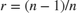punctured code is bounded above by the expression: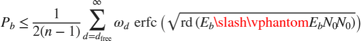In this expression, erfc denotes the complementary error function,is the code rate, and both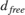and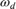are dependent on the particular code. For the rate 3/4 code of this example,= 5,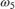= 42,= 201,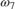= 1492, and so on. For more information, see reference [ 1 ].

Compute an approximation of the theoretical bound using the first seven terms of the summation for Eb/N0 values in 2:0.02:5. The values used for nerr come from reference [ 2 ], Table II.

Plot the simulation results, a fitted curve, and theoretical bounds.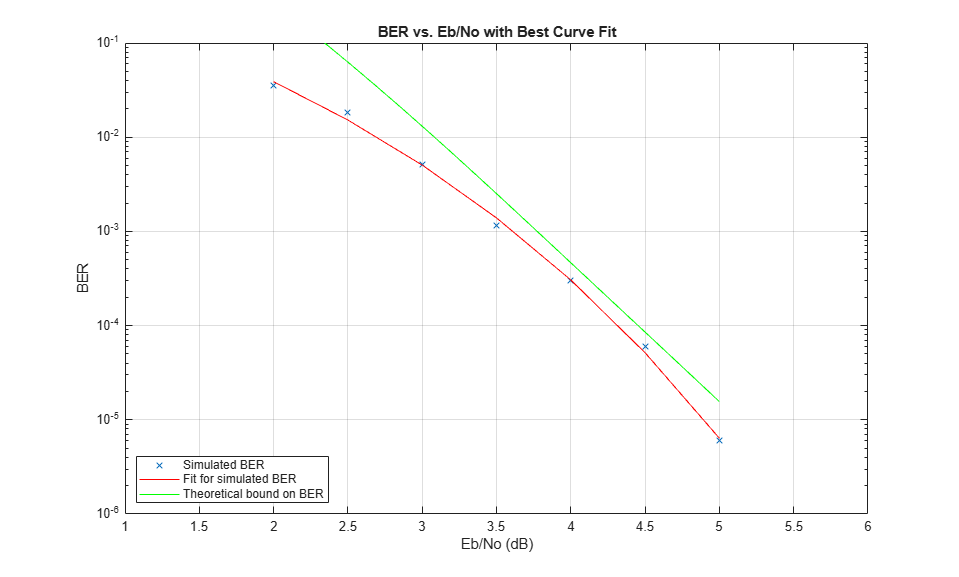In some cases, at the lower bit error rates, you might notice simulation results that appear to indicate error rates slightly above the bound. This result can come from the finite traceback depth in the decoder or, if you observe fewer than 500 bit errors, from simulation variance.

For an example that shows convolutional coding without puncturing, see the Soft-Decision Decoding section in Error Detection and Correction.

### References

1. Yasuda, Y., K. Kashiki, and Y. Hirata, "High Rate Punctured Convolutional Codes for Soft Decision Viterbi Decoding," IEEE Transactions on Communications, Vol. COM-32, March, 1984, pp. 315–319.

2. Begin, G., Haccoun, D., and Paquin, C., "Further results on High-Rate Punctured Convolutional Codes for Viterbi and Sequential Decoding," IEEE Transactions on Communications, Vol. 38, No. 11, November, 1990, p. 1923.